×

Search anything:

# Using MathML (math tag) in HTML

#### Software Engineering Web Development HTMLGet this book -> Problems on Array: For Interviews and Competitive Programming

MathML is a browser feature to support rendering of mathematical equations. It was the first approach towards serving mathematical equations on the web and is fully supported in FireFox. Currently, we have other approaches like MathJax which takes a different approach to solve this problem.

In this article, we will explore MathML and see how we can use it to render Mathematical equations on our HTML pages.

The MathML element is used to include mathematical expressions and equations in an HTML page. MathML is a powerful tool used to describe a various math expressions which you can create using common word processing packages, in addition to this it is suitable for rendering to speech as well. When rendering to text-only media, simple text graphics can be used to create math symbols such as integration sign, differentiation sign, etc. while other simpler symbols can be called by using their entity names, like the plus sign can be used by typing '+' sign directly from the keyboard.

MathML has two parts:

• Presentation MathML
• Content MathML

Presentation MathML is concerned with elements that defined a mathematical notation while Content MathML is concerned with how to handle the elements of Presentation MathML together.

### Presentation MathML

Presentation MathML plays the role of displaying equations on the HTML page and has around 30 elements associated with it. The elements' names all begin with the letter 'm'. A Presentation MathML expression is built from tokens in combination with higher level elements, which control their layout.

Token elements generally only contain characters like the following:

1. <mi>x</mi> – identifiers;
2. <mo>+</mo> – operators;
3. <mn>2</mn> – numbers.
4. <mtext>non zero</mtext> – text.

The above token elements are combined with layout elements, that generally contain only elements such as:

1. <mrow> – a horizontal row of items;
2. <msup>, <munderover> , and others – superscripts, limits over and under operators like sums, etc.;
3. <mfrac> – fractions;
4. <msqrt> and <mroot> – roots;
5. <mfenced> - surrounding content with fences, such as parentheses.

### Content MathML

Content MathML focuses on the semantics of the expression rather than its layout. Content MathML's primary element is the apply element that represents function application. The function is applied to the first child element under the apply tag, and its operands are the remaining child elements. Content MathML uses only a few attributes.

For example,

<apply><sin/><ci>x</ci></apply> represents sin(x) and
<apply><plus/><ci>x</ci><cn>5</cn></apply> represents x+5


The elements representing operators and functions are empty elements, because their operands are the other elements under the apply tag.

### Attributes

Below are some of the common attributes associated with MathML.

1. mrow - renders as a horizontal row containing its arguments.
<mrow>
<mn> 1 </mn>
<mo> + </mo>
<mn> 1 </mn>
</mrow>


Output:

1+1

1. mfenced - provides the possibility to add custom opening and closing parentheses and separators to an expression.
<mfenced open="{" close="}" separators=";;,">
<mi>a</mi>
<mi>b</mi>
<mi>c</mi>
<mi>d</mi>
<mi>e</mi>
</mfenced>


Output:

{a;b;c,d,e}

1. mfrac - used to display fractions.
<mfrac bevelled="true">
<mfrac>
<mi> a </mi>
<mi> b </mi>
</mfrac>
<mfrac>
<mi> c </mi>
<mi> d </mi>
</mfrac>
</mfrac>


Output:

(a/b)/(c/d)

1. msup - used to attach a superscript to an expression.
<msup>
<mi>X</mi>
<mn>2</mn>
</msup>


Output: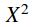1. mtable - allows you to create tables or matrices. Inside a mtable only mtr and mtd tags elements may appear.
<mtable frame="solid" rowlines="solid" align="axis 3">
<mtr>
<mtd><mi>A</mi></mtd>
<mtd><mi>B</mi></mtd>
</mtr>
<mtr>
<mtd><mi>C</mi></mtd>
<mtd><mi>D</mi></mtd>
</mtr>
<mtr>
<mtd><mi>E</mi></mtd>
<mtd><mi>F</mi></mtd>
</mtr>
</mtable>


Output: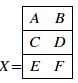### Examples

Each nested mrow is used to represent a separate term. In this example, the left hand side of the equation acts as one function to the operand "=" and the right hand side acts as a different function. Differentiating these terms helps in spacing for visual rendering, voice rendering, and line breaking. The ⁢ MathML character entity is used to indicate that there are special spacing rules between the 4 and x, and that the 4 and x should not be broken onto separate lines, instead they should be combined as a single term.

<mrow>
<mrow>
<msup>
<mi>x</mi>
<mn>2</mn>
</msup>
<mo>+</mo>
<mrow>
<mn>4</mn>
<mo>&InvisibleTimes;</mo>
<mi>x</mi>
</mrow>
<mo>+</mo>
<mn>4</mn>
</mrow>
<mo>=</mo>
<mn>0</mn>
</mrow>


Output-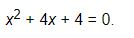### Solution to a quadratic equation in MathML

The mfrac and msqrt elements are used for generating fractions and square roots respectively. The "plus or minus" sign is represented by the entity name ± and equivalently you can use the character reference &#00B1;. We once again see the ⁢ for the term 4ac, to indicate that they are together as a single term.

<mrow>
<mi>x</mi>
<mo>=</mo>
<mfrac>
<mrow>
<mrow>
<mo>-</mo>
<mi>b</mi>
</mrow>
<mo>&PlusMinus;</mo>
<msqrt>
<mrow>
<msup>
<mi>b</mi>
<mn>2</mn>
</msup>
<mo>-</mo>
<mrow>
<mn>4</mn>
<mo>&InvisibleTimes;</mo>
<mi>a</mi>
<mo>&InvisibleTimes;</mo>
<mi>c</mi>
</mrow>
</mrow>
</msqrt>
</mrow>
<mrow>
<mn>2</mn>
<mo>&InvisibleTimes;</mo>
<mi>a</mi>
</mrow>
</mfrac>
</mrow>


Output-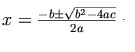### 2 x 2 Matrix in MathML

The mtable element indicates that a MathML table is being created. The mtr tag specifies a row of the table and the mtd tag holds the data for an element of a row. Most elements have a number of attributes that control the details of their screen and print rendering. For example, there are several attributes for the mfenced element that controls what delimiters should be used at the beginning or at the end of a grouped expression.

<mrow>
<mi>A</mi>
<mo>=</mo>
<mfenced open="[" close="]">
<mtable>
<mtr>
<mtd><mi>x</mi></mtd>
<mtd><mi>y</mi></mtd>
</mtr>
<mtr>
<mtd><mi>z</mi></mtd>
<mtd><mi>w</mi></mtd>
</mtr>
</mtable>
</mfenced>
</mrow>


Output-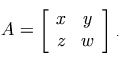### Integration in MathML

In this example, we use the semantics element to provide a MathML content expression to serve as a semantic annotation for a presentation expression. In the code below, we have used the msubsup tag to attach a subscript and a superscript to an expression, which is the integral sign for this example. We also used entities ∫ and ⅆ to specify the integral and differential symbols.

<mrow>
<semantics>
<mrow>
<msubsup>
<mo>&int;</mo>
<mn>1</mn>
<mi>t</mi>
</msubsup>
<mfrac>
<mrow>
<mo>&dd;</mo>
<mi>x</mi>
</mrow>
<mi>x</mi>
</mfrac>
</mrow>
<annotation-xml encoding="MathML-Content">
<apply>
<int/>
<bvar><ci>x</ci></bvar>
<lowlimit><cn>1</cn></lowlimit>
<uplimit><ci>t</ci></uplimit>
<apply>
<divide/>
<cn>1</cn>
<ci>x</ci>
</apply>
</apply>
</annotation-xml>
</semantics>
</mrow>


Output-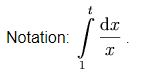### Web Browser Support

MathML is a low level tool and slightly outdated. It is only compatible in some browsers, meaning in other browsers, the format of mathematical equations will lose precision and style. Fortunately, tools exist which help you to get MathML working in unsupported browsers as well.

1. Firefox- FireFox has MathML support built in, but you need to ensure that your document is being served in the right way. In particular, you need to use XHTML so make sure you have the outputXHTML attribute on the htmlFilter element set to true within your EditLive! configuration.

2. Internet Explorer- Internet Explorer doesn’t have support for MathML built in, but you can use the MathPlayer plugin from Design Science to enable support.

3. Chrome- Chrome does not support MathML. Instead you have to use MathJax which can render both Presentation and Content MathML.

4. Opera- Recent versions of Opera have built in support for MathML in XHTML documents. Following the Authoring MathML for Mozilla should also make the document work with Opera.#### Abhinav Prakash

Intern at 1LearnApp, Hoopstop, Harvesting and OpenGenus | Bachelor's degree (2016 to 2020) in Computer Science at University of Massachusetts, Amherst

Using MathML (math tag) in HTML